Courses

# Salt Hydrolysis - Ionic Equilibrium Chemistry Notes | EduRev

## Physical Chemistry

Created by: Asf Institute

## Chemistry : Salt Hydrolysis - Ionic Equilibrium Chemistry Notes | EduRev

The document Salt Hydrolysis - Ionic Equilibrium Chemistry Notes | EduRev is a part of the Chemistry Course Physical Chemistry.
All you need of Chemistry at this link: Chemistry

Salt Hydrolysis:

1.  Hydrolysis of salt of [SA – SB]:

• Solution is neutral in nature (pH = pOH = 7)

• Ex NaCl, Na2SO4, KNO3 etc.

2.  Hydrolysis of [WA - SB]

• In this type of salt hydrolysis anion reacts with water therefore called as anionic hydrolysis.

• Solution is basic in nature as [OH] increases.

• pH of solution is greater than 7.

1. Relation between kh, kw, ka

CN + H2O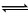HCN + OH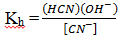…1

For weak acid, HCNH+ + CN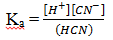… 2

For water,    H2OH+ + OH

Kw = [H+] [OH]                                            … 3

eq (1) x eq. (2) = eq (3)

kb × ka= kw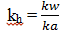b)  Degree of hydrolysis

CN + H2OHCN + OH

C                         0         0

C- Ch                Ch     Ch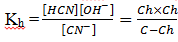Since h <<< 1

1 – h ≈ 1

Hence, kh = Ch2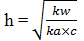c)   pH of the solution

[OH]  = Ch

[OH] =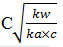[OH] =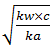Kw = [OH] [H+]

[H+] =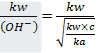[H+] =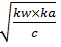On taking log both sides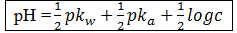3) Hydrolysis of strong Acid – Weak Base [SA - WB]

NH4Cl, (NH4)2 SO4 ZnCl2 etc.

In this type of salt hydrolysis, cation reacts with H2O, therefore called as cationic hydrolysis.

Solution is acidic in nature as [H+] is increased.

pH of the solution is less than 7.

a)   Relation between kh, kw and kb

NH4+ + H2ONH4OH + H+

Hydrolysis constant, kh =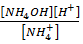…1

For weak base, NH4OHNH4+ + OH

Kb =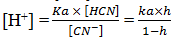… 2

For water, H2OH+ + OH

Kw = [H+] [OH]                                                        … 3

Multiply eq. (1) x (2) = eq. 3

i.e. kh x kb = kw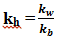b) Degree of hydrolysis – represented by h

NH4+ + H2ONH4OH + H+

C                           0            0

C – Ch                  Ch         Ch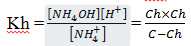Since h <<< 1, then (1 – h) ≈ 1

Kh = Ch2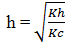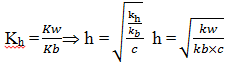c)   pH of the solution

pH = - log [H+]

[H+] = Ch =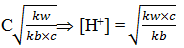On taking – log on both sides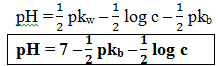4. Hydrolysis of [WA – WB]

ex: NH4CN, (NH4)2 CO3 etc.

Solution may be neutral, acidic or basic depending upon the nature of acid & base.

a) Relation between Kn1Kw1Ka and Kb

NH4+ + CN + H2ONH4OH + HCN

Kb =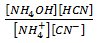… 1

For weak base, NH4OHNH4++OH

Kb =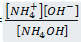…2

For weak acid, HCNH+ + CN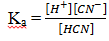For water, H2OH+ + OH

Kw = [OH] [H+]

Multiply eq. (1) ×eq (2) ×eq (3) = eq (4)

## Kh x kb x ka = kw

a) Degree of Hydrolysis

NH4+ + CN + H2ONH4OH + HCN

C        C                            0               0

At equilibrium             C – Ch C – Ch                   Ch              Ch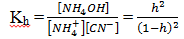Since, h <<< 1, then (1 - h) ≈ 1

Kh = h2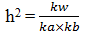b) pH of the solution

for eq. (3)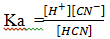[H+] =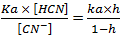Since, h <<< 1, then (1-h)  ≈ 1

[H+] = ka× h = ka×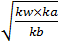Taking log both sides

- log [H+] =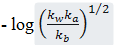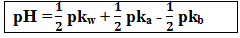Case I: If pka = pkb

Then pH = 7, the solution is neutral

Case II: If pKa>pkb

Then pH > 7, then solution is Basic

Case III: pKa<pKb

Then, pH < 7, solution is acidic

Example: what is the pH of 0.4 M aqueous solution of NaCN, given that the pkb (CN) is .70.

Solution:         CN + H2OHCN + OH

C                        O         O

C – Ch               Ch       Ch

pKb(CN) is given means equilibrium constant of this reaction is given. Because this reaction its express the basicity of CN ion.

pkb = - log kb

4.7 = - log kb

Kb = 1.9 × 10–5

now, kb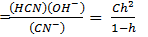since h <<< 1, the (1 – h) ≈ 1

kb = Ch2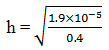h = 6.89 × 103

[OH] = Ch = 0.4 × 6.89 × 103= 2.76 × 103

pOH = - log [2.76 × 103]

pOH = 2.55

pH = 11.45

Example : Find out the kh of a centimolar solution of NH4Cl. If the dissociation constant for NH4OH is 106 and kw = 1014. Find the degree of hydrolysis and pH of solution.

Solution: NH4Cl is a salt of strong acid and weak base.

NH4+ + H2ONH4OH + H+

C                            0            0

C- Ch                   Ch        Ch

Equilibrium constant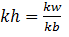Kb = 106

Kh =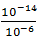=108

Kh = Ch2

108 = Ch2

[H+] = Ch = 102× 103 = 105

pH = 5

Dissociation of Polyprotic Acid

H3AH+        +          H2A'                            ka1

C- x                 x+y+z              x – y

H2AHA2–    +          H+                                          ka2

x-y                   y – z                x+y+z

HA2–A3–       +          H+                                          ka3

y-z                   z                      x+y+z

assumption:     ka1>> ka2>>> ka3

hence, x + y + z ≈ x

x – y≈ x

y - z≈ y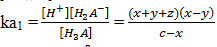So, Ka1 =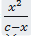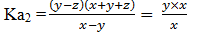Ka2 = y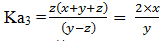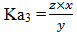• From eq1., you can calculate the value of x.

• By putting value of x you will get the value of y from eq. 2

• By putting values of x and y, you will get the value of 2 from eq. 3.

##### Hydrolysis of amphiprotic Anion:

This is one of the case of Anion hydrolysis, cations are not hydrolysed in this case.

NaHCO3etc. can undergo ionisation to form H+ ion and can undergo hydrolysis to form OH (Na+ ion is not hydrolysed)

Ionization

a)  1)   HCO3 + H2OCO32 +H3O+

acid

Hydrolysis

HCO3 + H2OH2CO+ OH

Base

pH (HCO3)    =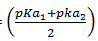Offer running on EduRev: Apply code STAYHOME200 to get INR 200 off on our premium plan EduRev Infinity!

## Physical Chemistry

77 videos|83 docs|32 tests

,

,

,

,

,

,

,

,

,

,

,

,

,

,

,

,

,

,

,

,

,

;